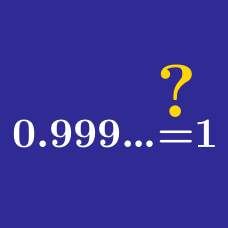Algebra

# Exponents Misconceptions

$\big(x^2\big)^3 =\, ?$

If $x^5 = 3$, then $(2x)^5 =$

If $x = 5$, then $\sqrt{x^2} =$

Which of the following is equal to $2^{-3}\text{?}$

For which choices of $a$ and $b$ is the equation $(ab)^\frac{1}{2} = a^\frac{1}{2}\cdot b^\frac{1}{2}$

not true?

×